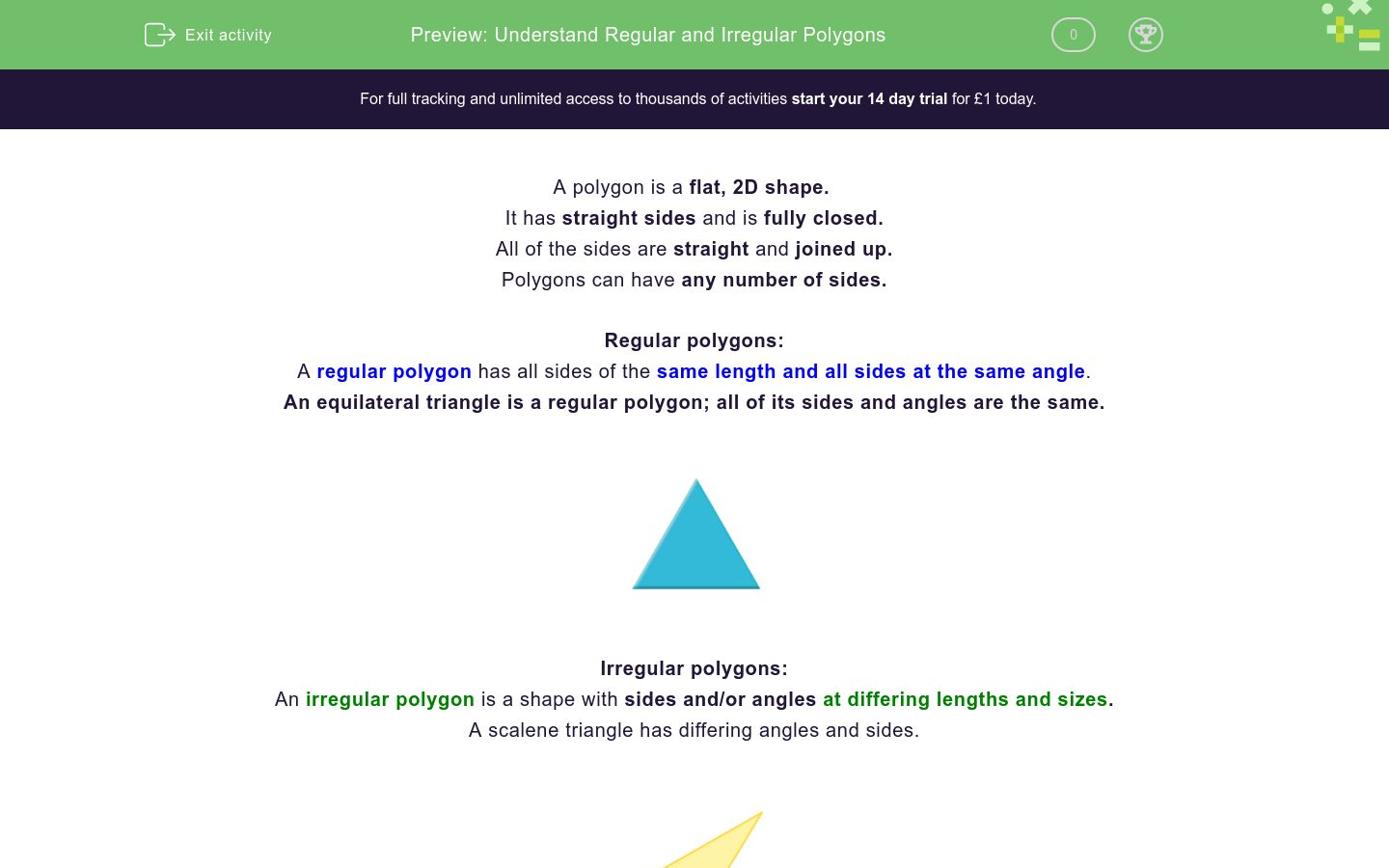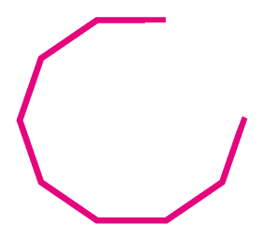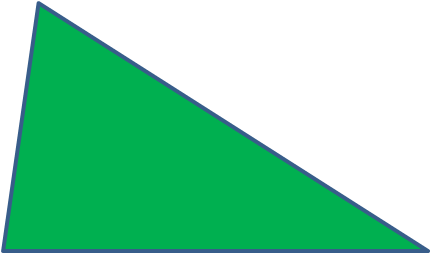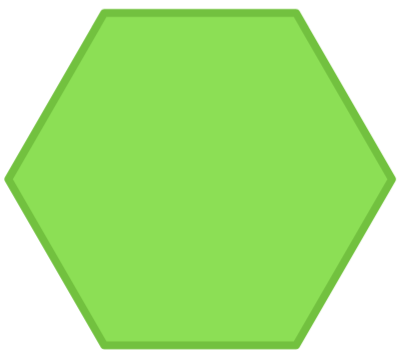# Understand Regular and Irregular Polygons

In this worksheet, students will be asked to build upon their existing knowledge of polygons to solve a variety of problem based questions.Key stage:  KS 2

Curriculum topic:   Geometry: Properties of Shapes

Curriculum subtopic:   Understand Polygons

Difficulty level:### QUESTION 1 of 10

A polygon is a flat, 2D shape.

It has straight sides and is fully closed.

All of the sides are straight and joined up.

Polygons can have any number of sides.

Regular polygons:

A regular polygon has all sides of the same length and all sides at the same angle.

An equilateral triangle is a regular polygon; all of its sides and angles are the same.Irregular polygons:

An irregular polygon is a shape with sides and/or angles at differing lengths and sizes.

A scalene triangle has differing angles and sides.Look at the shapes shown below.

Choose which shape is an irregular polygon.

Rectangle                                    DecagonRotated Square                      Equilateral triangleLook at the shapes shown below.

Can you correctly match the statements about the shapes?

Right Arrow                              DecagonRotated Square                      Equilateral triangle## Column B

Equilateral triangle
All of the sides and angles are equal
Rotated square
This regular polygon has 10 sides
Decagon
This shape can also be called a quadrilateral
Right arrow
This shape is irregular and the odd one out

Laura states that the semicircle below is a regular polygon.

Is she correct or incorrect?Correct

Incorrect

Is this shape a polygon?

Type yes or no.Is this shape a polygon?

Type yes or no.Is a 2D shape Is a 3D shape Has straight sides Is a closed shape (no gaps) Include open shapes Correct statements about polygons

Look at the polygons below and write the name of the regular polygon.Look at the shapes below and write the name of the irregular polygon.Lydia stated,

"A square is a rectangle."

Is Lydia correct or incorrect?

Incorrect

Correct

Harry states,

"A rhombus is a parallelogram."

Is Harry correct or incorrect?

Incorrect

Correct

• Question 1

Look at the shapes shown below.

Choose which shape is an irregular polygon.

Rectangle                                    DecagonRotated Square                      Equilateral triangleEDDIE SAYS
The rectangle is the irregular polygon as it has 4 equal angles but the sides are of differing lengths. How did you find this question? Don't worry we have more to for you to practise so that you get really confident!
• Question 2

Look at the shapes shown below.

Can you correctly match the statements about the shapes?

Right Arrow                              DecagonRotated Square                      Equilateral triangle## Column B

Equilateral triangle
All of the sides and angles are e...
Rotated square
This shape can also be called a q...
Decagon
This regular polygon has 10 sides
Right arrow
This shape is irregular and the o...
EDDIE SAYS
How did you get on matching the statements correctly? The equilateral triangle, rotated square and decagon are all regular polygons with equal sides and angles. The right arrow is irregular, as it doesn't have equal sides or angles that join the sides.
• Question 3

Laura states that the semicircle below is a regular polygon.

Is she correct or incorrect?Incorrect
EDDIE SAYS
Laura is incorrect, a shape with curved sides is not a polygon. A polygon must have straight sides only.
• Question 4

Is this shape a polygon?

Type yes or no.No
EDDIE SAYS
No, this shape is not a polygon as it has sides that intersect. A polygon cannot have intersecting sides. You've got this, keep going.
• Question 5

Is this shape a polygon?

Type yes or no.No
EDDIE SAYS
No, this shape is not a polygon as it has sides that are open. A polygon cannot have any gaps in its sides.
• Question 6

 Is a 2D shape Is a 3D shape Has straight sides Is a closed shape (no gaps) Include open shapes Correct statements about polygons
EDDIE SAYS
How did you get on identifying the properties of 2D shapes? The correct statements are: Is a 2D shape. Has straight sides. Is a closed shape (no gaps). Are you finding this easier as you move through the questions?
• Question 7

Look at the polygons below and write the name of the regular polygon.Square
EDDIE SAYS
The only regular polygon shown is the square. The scalene triangle, pentagon and rhombus are all irregular polygons.
• Question 8

Look at the shapes below and write the name of the irregular polygon.Trapezium
EDDIE SAYS
The irregular polygon is the trapezium. The regular polygons are The rotated square Equilateral triangle Hexagon This question was a little more challenging so don't worry if you got it wrong!
• Question 9

Lydia stated,

"A square is a rectangle."

Is Lydia correct or incorrect?

Correct
EDDIE SAYS
Yes, Lydia is correct. A square is a special type of rectangle. It is a special type of rectangle that has all sides the same length.
• Question 10

Harry states,

"A rhombus is a parallelogram."

Is Harry correct or incorrect?

Correct
EDDIE SAYS
Harry is correct, a rhombus is a parallelogram. A rhombus is a parallelogram because opposite sides are parallel and opposite angles are equal (the same as a parallelogram).
---- OR ----

Sign up for a £1 trial so you can track and measure your child's progress on this activity.

### What is EdPlace?

We're your National Curriculum aligned online education content provider helping each child succeed in English, maths and science from year 1 to GCSE. With an EdPlace account you’ll be able to track and measure progress, helping each child achieve their best. We build confidence and attainment by personalising each child’s learning at a level that suits them.

Get started